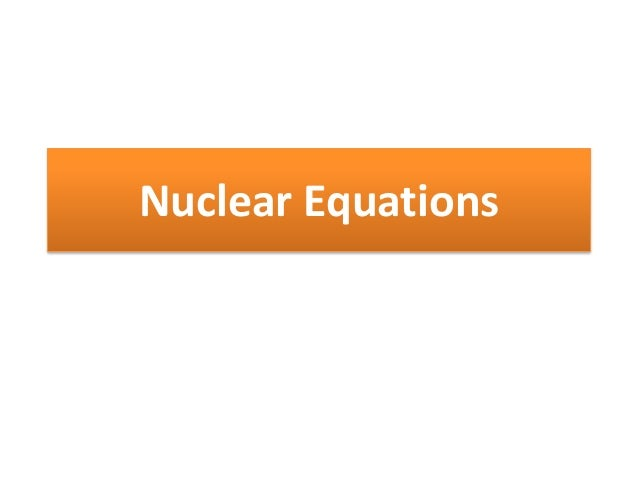Successfully reported this slideshow.
We use your LinkedIn profile and activity data to personalize ads and to show you more relevant ads. You can change your ad preferences anytime.Upcoming SlideShare
Loading in …5
×

# Nuclear equations

How to write nuclear equations for Option D IB Chemistry.

Taken from www.houstonisd.org/cms/lib2/TX01001591/.../13%20nuclear.pptx

• Full Name
Comment goes here.

Are you sure you want to Yes No
Your message goes here• Login to see the comments

• Be the first to like this

### Nuclear equations

1. 1. Nuclear Equations
2. 2. Nuclear Reactions • Nuclear reactions are different from chemical reactions Chemical Reactions Mass is conserved (doesn’t change) Small energy changes No changes in the nuclei; involve ONLY valance electrons Nuclear Reactions Small changes in mass Huge energy changes protons, neutrons, electrons and gamma rays can be lost or gained
3. 3. BALANCING NUCLEAR EQUATIONS 1. The sums of mass numbers (left superscripts) on each side must be equal. 2. The sums of atomic numbers or nuclear charges (left subscripts) on each side of the equation must be equal. Examples: 238U 4He + 234Th 92 2 90 214Pb 0 b- + 214Bi 82 1 83 3. Charges are not included in nuclear equations except to distinguish between the two types of b particles
4. 4. Balancing Nuclear Equations Complete the following nuclear equations: 1. 217At 213Bi + ? 2. 231Th 0b- + ? 1 3. 208Tl 0b- + ? 1
5. 5. Balancing Nuclear Equations Complete the following nuclear equations: 1. 217At 213Bi + 2. 231Th 0b- + 1 3. 208Tl 0b- + 1 85 83 90 81